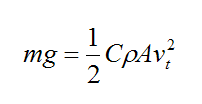Search

# The science Behind Skydiving- PART 1

INTRODUCTION

Skydiving may seem a very exciting and fun sport to pursue. But before jumping out from 15,000 feet above sea level and pulling the parachute, it is necessary to completely understand how the mechanism of skydiving works and what is the science behind it. As a matter of fact, one cannot become a verified professional skydiver, until one passes the written and oral A license exam; it covers the fundamental concepts of mechanical properties of fluids and solids. This proves how complex of a topic skydiving can really be if a person decides to pursue it professionally.

SKYDIVING AS A SPORT

Skydiving is one of the most thrilling and hard- to-pursue sport. It requires a very physically and mentally fit body. The bracket of people who can actually pursue this sport is very thin. For example, people with chronic diseases are not recommended to do it, as well as people with breathing and heart diseases should always be kept away from such activity, because of THE limited supply of oxygen at higher altitudes. The point is that one has to maintain a high level of fitness, if one actually wants to pursue this sport, either as a hobby or professionally.

BASIC CONCEPT

Before getting into the depth of the concept, it is important to understand that when a person is skydiving, he is a freely falling object, and therefore, there are a lot of forces acting in the person skydiving. As soon as the person jumps out of the chopper, he starts to accelerate towards the ground at a pretty high velocity. The main concept to understand here is the relation/interaction between the terminal velocity and gravitational pull.

WHAT IS TERMINAL VELOCITY

Terminal velocity can be defined as the maximum velocity attainable by an object as it falls through a fluid. It occurs when the sum of the drag force is equal to the downward force of gravity acting on the object. Since the net force on the object is zero, the object has zero acceleration.

When the person is falling downwards, there comes a moment, when his acceleration becomes zero.

The FBD (free body diagram) of the body can be drawn as follows-RELATIONSHIP BETWEEN TERMINAL VELOCITY AND GRAVITATIONAL PULL

According to laboratory calculations and researches, the general equation of drag force acting on a body can be given by-

D=reference area/2 X density X square of velocity X drag coefficient

It can also be written as the following-{Equation 1}

In order to derive the equation for the Relationship between terminal velocity and gravitational force, we also need to consider the gravitational force i.e. W=mg.

In the last heading, we had defined terminal velocity. Taking reference from that paragraph, we know D=W, where D is the drag force applied on the body and W is the gravitational force.

From the first two equations, we can write-{Equation 2}

Where-

C is the drag coefficient, which can vary along with the speed of the body.

ρ is the density of the fluid through which the body is moving

v is the speed of the body relative to the fluid

A is the projected cross-sectional area of the body perpendicular to the flow direction

M is the mass of the body.

g is 9.8m/s^2.

We can now obtain the formula for terminal velocity as -{Equation 3}

HOW DO SKYDIVERS CONTROL THEIR MOTION IN THE AIR?

Some of the professional skydivers are able to control their movement while they are in the air. This is not simply because of the change in air pressure, but it is due to the change in the terminal velocity of the skydiver. As we can see in equation #3, terminal velocity is indirectly proportional to the area of cross-section, and therefore, change in the body shape leads to a change in terminal velocity and ultimately their motion.

MORE ON THIS TOPIC IN THE NEXT POST. Part 2 coming soon.